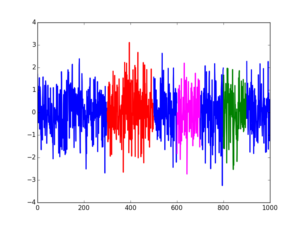Select PageOften times, we need to overlay colors on segments of a time-series or other line-plot. The colors could be indicative of some events or to highly particularly important time-spans of interest. Here’s a short function that can be used to generate such line segments of multiple colors using matplotlib in python.

```#!/usr/bin/env python
# Author: Abhay Harpale

"""
plot multicolored lines in matplotlib
"""

import matplotlib.pyplot as plt
import numpy as np

def find_contiguous_colors(colors):
# finds the continuous segments of colors and returns those segments
segs = []
curr_seg = []
prev_color = ''
for c in colors:
if c == prev_color or prev_color == '':
curr_seg.append(c)
else:
segs.append(curr_seg)
curr_seg = []
curr_seg.append(c)
prev_color = c
segs.append(curr_seg) # the final one
return segs

def plot_multicolored_lines(x,y,colors):
segments = find_contiguous_colors(colors)
plt.figure()
start= 0
for seg in segments:
end = start + len(seg)
l, = plt.gca().plot(x[start:end],y[start:end],lw=2,c=seg)
start = end
```

Here is some code to generate an example plot using this:

```x = np.arange(1000)
y = np.random.randn(1000) # randomly generated values
# color segments
colors = ['blue']*1000
colors[300:500] = ['red']*200
colors[800:900] = ['green']*100
colors[600:700] = ['magenta']*100

plot_multicolored_lines(x,y,colors)
plt.show()
```